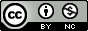This document was produced using pomp version 3.1.1.0.

Iterated filtering is a technique for maximizing the likelihood obtained by filtering. In pomp, it is the particle filter that is iterated. The iterated filtering of E. L. Ionides, Bretó, and King (2006) is implemented in the mif function. Edward L. Ionides, Nguyen, Atchadé, Stoev, and King (2015) describe an improvement on the original (E. L. Ionides et al. 2006) algorithm. This "IF2" algorithm is implemented in the mif2 function.

## IF2 algorithm pseudocode

model input: Simulators for $$f_{X_0}(x_0;\theta)$$ and $$f_{X_n|X_{n-1}}(x_n| x_{n-1}; \theta)$$; evaluator for $$f_{Y_n|X_n}(y_n| x_n;\theta)$$; data, $$y^*_{1:N}$$

algorithmic parameters: Number of iterations, $$M$$; number of particles, $$J$$; initial parameter swarm, $$\{\Theta^0_j, j=1,\dots,J\}$$; perturbation density, $$h_n(\theta|\varphi;\sigma)$$; perturbation scale, $$\sigma_{1{:}M}$$

output: Final parameter swarm, $$\{\Theta^M_j, j=1,\dots,J\}$$

1. $$\quad$$ For $$m$$ in $$1{:} M$$
2. $$\quad\quad\quad$$ $$\Theta^{F,m}_{0,j}\sim h_0(\theta|\Theta^{m-1}_{j}; \sigma_m)$$ for $$j$$ in $$1{:} J$$
3. $$\quad\quad\quad$$ $$X_{0,j}^{F,m}\sim f_{X_0}(x_0 ; \Theta^{F,m}_{0,j})$$ for $$j$$ in $$1{:} J$$
4. $$\quad\quad\quad$$ For $$n$$ in $$1{:} N$$
5. $$\quad\quad\quad\quad\quad$$ $$\Theta^{P,m}_{n,j}\sim h_n(\theta|\Theta^{F,m}_{n-1,j},\sigma_m)$$ for $$j$$ in $$1{:} J$$
6. $$\quad\quad\quad\quad\quad$$ $$X_{n,j}^{P,m}\sim f_{X_n|X_{n-1}}(x_n | X^{F,m}_{n-1,j}; \Theta^{P,m}_{n,j})$$ for $$j$$ in $$1{:} J$$
7. $$\quad\quad\quad\quad\quad$$ $$w_{n,j}^m = f_{Y_n|X_n}(y^*_n| X_{n,j}^{P,m} ; \Theta^{P,m}_{n,j})$$ for $$j$$ in $$1{:} J$$
8. $$\quad\quad\quad\quad\quad$$ Draw $$k_{1{:}J}$$ with $$P[k_j=i]= w_{n,i}^m\Big/\sum_{u=1}^J w_{n,u}^m$$
9. $$\quad\quad\quad\quad\quad$$ $$\Theta^{F,m}_{n,j}=\Theta^{P,m}_{n,k_j}$$ and $$X^{F,m}_{n,j}=X^{P,m}_{n,k_j}$$ for $$j$$ in $$1{:} J$$
10. $$\quad\quad\quad$$ End For
11. $$\quad\quad\quad$$ Set $$\Theta^{m}_{j}=\Theta^{F,m}_{N,j}$$ for $$j$$ in $$1{:} J$$
12. $$\quad$$ End For

## An example

The following constructs the Gompertz example that is provided with pomp (see ?gompertz) and extracts the parameters at which the data were generated. It is meant to be directly comparable with the example displayed in the King, Nguyen, and Ionides (2016) J. Stat. Softw. paper.

library(pomp)
gompertz() -> gomp
theta <- coef(gomp)
theta.true <- theta

Let's use IF2 to obtain an approximate MLE for these data. We'll initialize the algorithm at several starting points around theta.true and just estimate the parameters $$r$$, $$\tau$$, and $$\sigma$$:

library(foreach)
library(doParallel)
registerDoParallel()

estpars <- c("r","sigma","tau")
library(doRNG)
registerDoRNG(525386942)

foreach(i=1:10,.inorder=FALSE) %dopar% {
theta.guess <- theta.true
theta.guess[estpars] <- rlnorm(
n=length(estpars),
meanlog=log(theta.guess[estpars]),
sdlog=1
)
gomp %>%
mif2(
Nmif=50,
params=theta.guess,
rw.sd=rw.sd(r=0.02,sigma=0.02,tau=0.05),
cooling.fraction.50=0.95,
Np=2000
) %>%
continue(Nmif=50,cooling.fraction=0.8) %>%
continue(Nmif=50,cooling.fraction=0.6) %>%
continue(Nmif=50,cooling.fraction=0.2) -> m1
ll <- replicate(n=10,logLik(pfilter(m1,Np=10000)))
list(mif=m1,ll=logmeanexp(ll,se=TRUE))
} -> mf
lls <- sapply(mf,getElement,"ll")
best <- which.max(sapply(mf,getElement,"ll")[1,])
theta.mif <- coef(mf[[best]]\$mif)

replicate(10,logLik(pfilter(gomp,params=theta.mif,Np=10000))) %>%
logmeanexp(se=TRUE) -> pf.loglik.mif

Note that gompertz() uses parameter transformations to ensure that the search for the MLE is constrained to positive parameters. See the pomp documentation (?pomp) and the section on Parameter Transformations in the Getting Started vignette).

Each of the 10 mif2 runs ends up at a different point estimate. We focus on that with the highest estimated likelihood, having evaluated the likelihood several times to reduce the Monte Carlo error in the likelihood evaluation. The particle filter produces an unbiased estimate of the likelihood; therefore, we will average the likelihoods, not the log likelihoods.

Convergence plots can be used to help diagnose convergence of the iterated filtering algorithm. Something like the following can be obtained by executing plot(mf).